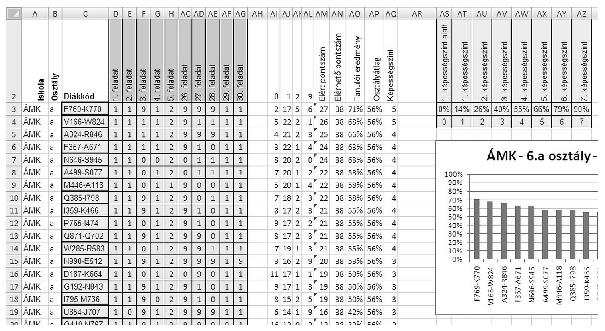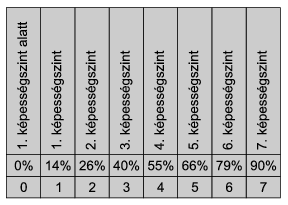Mathematical and Physical Journal
for High Schools
Issued by the MATFUND Foundation
 Already signed up? New to KöMaL?

#Problem I. 281. (December 2011)

I. 281. The table below contains students' results from some classes of four secondary schools in a natural science knowledge survey. There were 30 test questions. Each is evaluated by 0, 1, 2 and 9. The meaning of 0, 1 and 2 is the corresponding point obtained for that question, whereas 9 was given if a student scored 0 points because of not working on that problem at all.Create a spreadsheet i281, save it in the default file format of the application and evaluate the results according to the following (we hid some columns in the example so as to make the rightmost column also visible).

Auxiliary computations can be performed right to the below mentioned columns or below the data cells. If a value of a cell can not be computed, your program should replace it with suitable quantities, so the program will be able to solve further tasks depending on that result.

1. Open the text file term6_forras.txt (downloadable from our web page, in UTF-8 encoding) in a sheet Term6 beginning with cell A1. The first row of the sheet contains the maximal points for each exercise.

2. Insert the table header in the second row according to the example and hide the first row.

3. Write the evaluation codes in the second row of columns AI:AL. Rows below those should contain the number a particular student in that row got this mark in the header. These should be computed by functions, the formula in every column and row should be a copyable one.

4. Column AM should contain the total number of points of the student. The column header is Total points''.

5. Column AN should contain the maximal number of points that can be obtained by the students. The column header is Maximal points''.

6. The column header in column AO is Student's result'', below which results of each student should appear as an integer interpreted as percentages and computed from the previous two columns.

7. The column header in column AP is Class average''. The column contains his or her class average by using the same formula in that column.

8. Based on the results of the survey, each student is grouped into one of the 7 talent levels (képességszint''), or into a 0th level (1. képességszint alatt''). You should create a similar table in cells AS2:AZ4.9. Based on the table and the performance of the student, column AQ should contain the talent levels as integers between 0 and 7 for each student, by using the same formula throughout the column. (Talent level 3'' for example contains all students who scored at least 40%, but below 55%.) The column header should be Talent level''.

10. Sort the data according to the name of the school and class, and then into descending order according to student levels.

11. Create a bar chart similar to the example showing the data for the first class of the first school after the sorting. The title of the diagram should be the name of the school, then 6.'', then the name of the corresponding class, and the date of the survey, that is 2011 May.

The spreadsheet (i281.xls, i281.ods, ...) together with a short documentation (i281.txt, i281.pdf, ...) also describing the name and version number of the spreadsheet application should be submitted in a compressed file i281.zip.

(10 pont)

Deadline expired on January 10, 2012.

Sorry, the solution is available only in Hungarian. Google translation

Mintamegoldásként Adrián Patrik Debreceni 12. osztályos tanuló Access 2010-ben készül munkáját (i281.xlsx) és kitűnő dokumentációját (i281.pdf), valamint Kovács Balázs Marcell Budapesti 9. osztályos diák Libreoffice Calc-ban készült megoldását (i281.ods) közöljük.

### Statistics:

 10 students sent a solution. 10 points: Adrián Patrik, Barkaszi Richárd Miklós, Fényes Balázs, Gema Barnabás, Hoffmann Áron, Kovács Balázs Marcell, Kucsma Levente István. 9 points: Antal János Benjamin. 8 points: 1 student. 7 points: 1 student.

Problems in Information Technology of KöMaL, December 2011